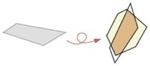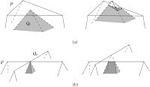Shape matching is an important ingredient in shape retrieval, recognition and classification, alignment and registration, and approximation and simplification. In a large database of shapes, for example, shape retrieval searches for all shapes similar to a query shape.

In geometric shape matching, we are given two geometric objects and compute the similarity of them based on some geometric similartity measure. In most cases, we transform one of them over the other, by scaling, translation, and rotation. Shape matching algorithms provide fundamental techniques for shape recognition and retrieval, and shape classification for large scaled shape databases. They are also used in shape alignment and registration.

#### Maximizing the Overlap of Two Planar Convex Sets under Rigid MotionsGiven two compact convex sets P and Q in the plane, we compute an image of P under a rigid motion that approximately maximizes the overlap with Q. More precisely, for any eps>0, we compute a rigid motion such that the area of overlap is at least 1-eps times the maximum possible overlap. Our algorithm uses O(1/eps) extreme point and line intersection queries on Pand Q, plus O((1/eps2)log(1/eps)) running time. If only translations are allowed, the extra running time reduces to O((1/eps)log(1/eps)). If P and Q are convex polygons with n vertices in total that are given in an array or balanced tree, the total running time is O((1/eps)logn+(1/eps2)log(1/eps))for rigid motions and O((1/eps)logn+(1/eps)log(1/eps)) for translations.

Hee-Kap Ahn, Otfried Cheong, Chong-Dae Park, Chan-Su Shin and Antoine Vigneron
In Proc. 21st Annu. ACM Symposium on Computational Geometry, 2005, and in Computational Geometry: Theory and Applications, 37(1):3-15, 2007

#### Stacking and Bundling two Convex PolygonsGiven two compact convex sets C1 and C2 in the plane, we consider the problem of finding a placement deltaC1 of C1 that minimizes the area of the convex hull of the union of deltaC1 andC2. We first consider the case where deltaC1 and C2 are allowed to intersect (as in “stacking” two flat objects in a convex box), and then add the restriction that their interior has to remain disjoint (as when “bundling” two convex objects together into a tight bundle). In both cases, we consider both the case where we are allowed to reorient C1, and where the orientation is fixed. In the case without reorientations, we achieve exact near-linear time algorithms, in the case with reorientations we compute a (1+eps)-approximation in time O(eps(-1/2)logn+ eps(-3/2)logeps(-1/2)), if two sets are convex polygons with n vertices in total.

Hee-Kap Ahn, Otfried Cheong
In Proc. 16th Annual International Symposium on Algorithms and Computation, pages 40-49, 2005.

#### Maximum Overlap and Minimum Convex Hull of Two Convex Polyhedra under TranslationsGiven two convex polyhedra P and Q in three-dimensional space, we consider two related problems of shape matching: (1) finding a translation t1 in R3 of Q that maximizes the volume of their overlap of P and (Q+t1), and (2) finding a translation t2 in R3 that minimizes the volume of the convex hull of P U (Q+t2). For the maximum overlap problem, we observe that the d-th root of the objective function is concave and present an algorithm that computes the optimal translation in expected time O(n3log4n). This method generalizes to higher dimensions d>3 with expected running time O(nd+1 -3/d(logn)d+1). For the minimum convex hull problem, we show that the objective function is convex. The same method used for the maximum overlap problem can be applied to this problem and the optimal translation can be computed in the same time bound.Hee-Kap Ahn, Peter Brass and Chan-Su Shin
Computational Geometry: Theory and Applications, 40, pages 171-177, 2008

#### Maximum Overlap of Convex Polytope under Translation

Given two convex polytopes bounded by a total of n hyperplanes in Rd for some constant d >= 3, we compute the translation of one in order to maximize the volume of the intersection with the other polytope. Under some mild non-degeneracy assumptions, our algorithm runs inO(n|_d/2_|+1logd-1 n) time with probability at least 1-n-O(1). The running time can be improved toO(nlog3 n) in three dimensions.

Hee-Kap Ahn, Siu-Wing Cheng and Iris Reinbacher